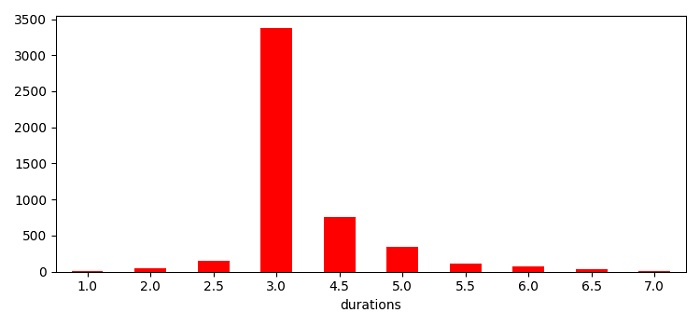# Plotting a cumulative graph of Python datetimes in Matplotlib

To plot a cumulative graph of python datetimes, we can take the following steps −

• Set the figure size and adjust the padding between and around the subplots.

• Make a Pandas dataframe with some college data, where one key for time difference and another key for number students have admissioned in the subsequent year.

• Plot the dataframe using plot() method where kind='bar', i.e., class by name.

• To display the figure, use show() method.

## Example

import pandas as pd
from matplotlib import pyplot as plt

plt.rcParams["figure.figsize"] = [7.50, 3.50]
plt.rcParams["figure.autolayout"] = True

college_student_data = {'durations': [1, 2, 2.5, 3, 4.5, 5, 5.5, 6, 6.5, 7], 'admissions': [5, 50, 150, 3380, 760, 340, 115, 80, 40, 10]}
df = pd.DataFrame(data=college_student_data)

df.plot(kind='bar', x='durations', y='admissions', legend=False, color='red', rot=0,)

plt.show()

## OutputUpdated on: 03-Jun-2021

444 Views